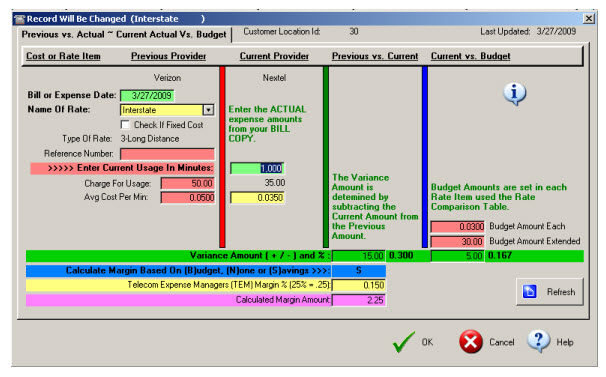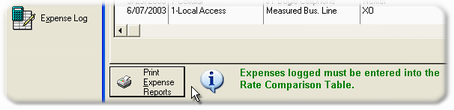﻿ Update Expense Log

# Update Expense LogExpense Log Edit Window

The Expense Log Editor and calculation window is very similar to the Rate Comparison calculator. Only the Type of Rates that appear in the Rate Comparison table may be entered into the Expense Log.

The Previous Provider column data is merged from the Rate Comparison entry. The user must enter the minutes of usage or the Qty of Fixed in the Current Provider column.

The Budget column is computed according to the minutes of usage or Qty of Fixed amount entered and multiplied by the Budget amount entered into the Rate Comparison table for a particular expense.

The Budget Variance Amount is automatically computed by the following formula:

(Extended Budget Amount - Extended Current Provided Amount)

The Budget Variance Percent is automatically computed by the following formula:

(Budget Variance Amount/Extended Budget Amount)

The Margin % must be entered into the Rate Comparison table.

The Margin Amount is computed by the following formula:

(Budget Variance Amount * Margin %)

The Expense Reports can only be called from the Browse the Expense Log table.